# FAQ¶

## What do I need to do to see the effect of a pulse sequence?¶

The simulation requires the definition of the spin system and its parameters (offset frequencies and coupling constants). Also make sure the desired initial state and the pulse sequence are specified. Then the simulation can be started with the play button and the DROPS display shows the effect on the state of the spin system in real time.

## How can I see what a particular operator looks like?¶

Simply define the operator of interest in the menu Initial State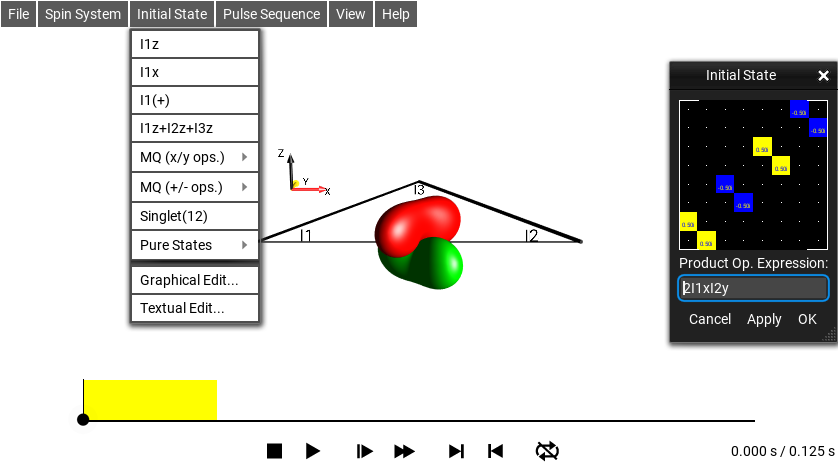Fig. 262 $$\rho_0 = 2I_{1x}I_{2y}$$

In this example, the operator $$2I_{1x}I_{2y}$$ is displayed.

(In the menu Initial State ‣ Textual Edit…, it is defined using the short-hand notation 2I1xI2y.)

• Tip 1: Before defining the operator, make sure the time slider is at the beginning of the selected pulse sequence. (In order to actually see the defined operator and not what it has evolved to during the pulse sequence.) A simple way to do this is to press thebutton.

• Tip 2: The triangle can be removed by setting all couplings to 0 Hz (open Spin System ‣ Parameters…). To hide the parameter sliders, just close the window.

## How can I see which product operators are represented by a given DROPS display?¶

To see the current list of product operators, select View ‣ List Prod. Ops…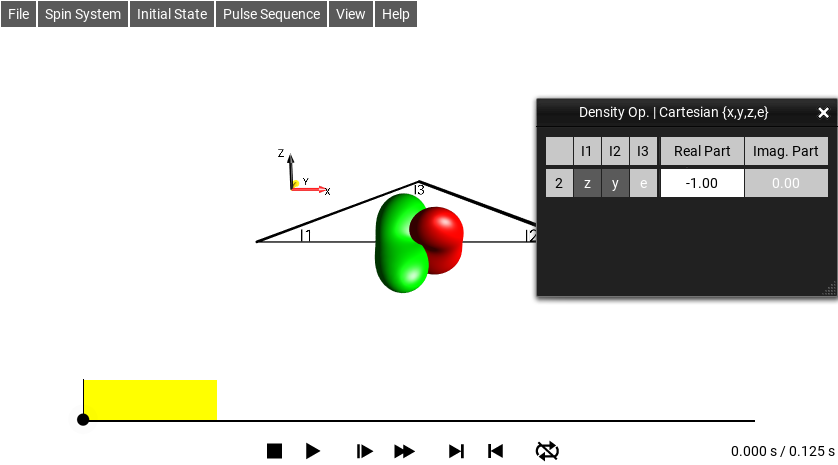Fig. 263 Cartesian Product Operator List

The Cartesian product operator terms are displayed using the three-letter (see short-hand notation).

In this example, the DROPS display shows −1 zye, which is the shorthand notation for the Cartesian product operator $$-2I_{1z}I_{2y}$$.

## How can I see (and modify) the current frequency offsets and coupling constants of the spins system?¶

Select Spin System ‣ Parameters…

The parameter sliders and the current values of the parameters are displayed in the System Parameters window.Fig. 264 Parameter Window

In this example, the offset frequencies of the first, the second, and the third spin are $$1 Hz$$, $$-1 Hz$$, and $$2 Hz$$, respectively.

The coupling constants are $$J12 = 2 Hz$$, $$J13 = 0.6 Hz$$, and $$J23 = -2 Hz$$.

• Tip 1 The relative size and sign of the coupling constants is represented by the thickness and color (positive: white; negative: yellow) of the lines connecting the corresponding spins.

## How do I choose the initial state of the spin system?¶

In the menu Initial State, a selection of initial states of interest is presented. In a typical experiments, you may be interested to start e.g. with z magnetization of the first spin of of all spins. Examples of operator you can select are given below:

Initial State ‣ I1z

z magnetization of the first spin

Initial State ‣ I1x

x magnetization of the first spin

Initial State ‣ I1(+)

+1-quantum coherence of the first spin

Initial State ‣ I1z + I2z + I3z

z magnetization of the first, the second, and the third spin

Initial State ‣ MQ(x/y ops.)

Multiple-quantum operators with quantum order (+p) AND (-p) based real combination of Cartesian product operators

Initial State ‣ MQ(+/- ops.)

Multiple-quantum operators with quantum order (+p) OR (-p) based on raising and lowering operators

Initial State ‣ Singlet(12)

The traceless part of the singlet state involving the first and second spin

Initial State ‣ Graphical Edit…

This option makes opens a window to edit the chosen initial density operator and generate any desired operator of interest

## Examples of States and Droplets of a Single Spin¶

DROPS state

Initial State ‣ I1z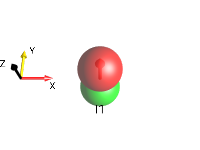Initial State ‣ I1x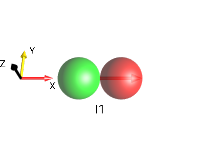Initial State ‣ I1y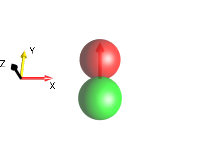Initial State ‣ I1(+)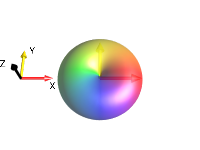Initial State ‣ I1(-)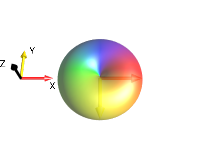## Examples of Zero-Quantum Coherences Involving Two Spins¶

DROPS state

Initial State ‣ MQ(x/y ops.) ‣ 0Q(I1,I2) ‣ 0Qx(I1,I2)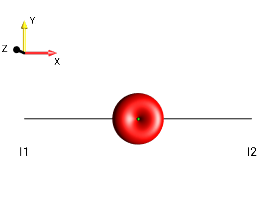zero-quantum x coherence $$I_{1x}I_{2x} + I_{1y}I_{2y}$$

Initial State ‣ MQ(x/y ops.) ‣ 0Q(I1,I2) ‣ 0Qy(I1,I2)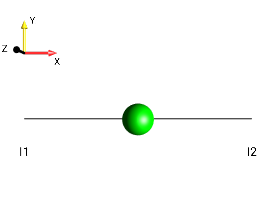zero-quantum y coherence $$I_{1y}I_{2x} - I_{1x}I_{2y}$$

Initial State ‣ MQ(+/- ops.) ‣ 0Q(I1,I2) ‣ I1(+)*I2(-)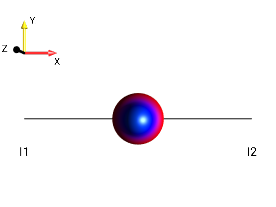$$I_{1}^{+}I_{2}^{-}$$

Initial State ‣ MQ(+/- ops.) ‣ 0Q(I1,I2) ‣ I1(-)*I2(+)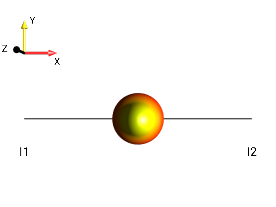$$I_{1}^{-}I_{2}^{+}$$

## Examples of Single-Quantum Coherences Involving Two Spins¶

DROPS state

Initial State ‣ MQ(x/y ops.) ‣ 1Q(I1,I2) ‣ 2*I1x*I2z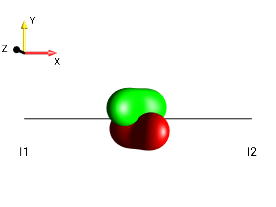antiphase x coherence $$2I_{1x}I_{2z}$$

Initial State ‣ MQ(x/y ops.) ‣ 1Q(I1,I2) ‣ 2*I1y*I2z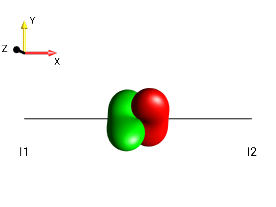antiphase y coherence $$2I_{1y}I_{2z}$$

Initial State ‣ MQ(+/- ops.) ‣ 1Q(I1,I2) ‣ I1(+)*I2z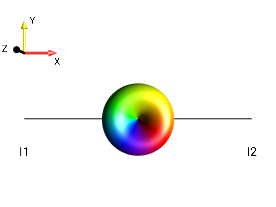+1 quantum antiphase coherence $$2I_{1}^{+}I_{2z}$$

Initial State ‣ MQ(+/- ops.) ‣ 1Q(I1,I2) ‣ I1(-)*I2z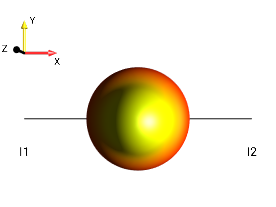−1 quantum antiphase coherence

## Examples of Double-Quantum Coherences Involving Two Spins¶

DROPS state

Initial State ‣ MQ(x/y ops.) ‣ 2Q(I1,I2) ‣ 2Qx(I1,I2)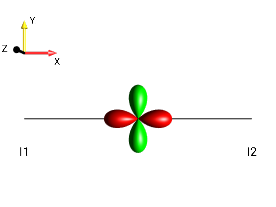double-quantum x coherence $$I_{1x}I_{2x} − I_{1y}I_{2y}$$

Initial State ‣ MQ(x/y ops.) ‣ 2Q(I1,I2) ‣ 2Qy(I1,I2)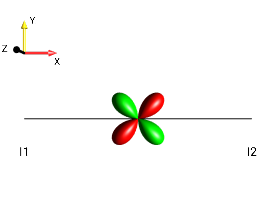double-quantum y coherence $$I_{1y}I_{2x} + I_{1x}I_{2y}$$

Initial State ‣ MQ(+/- ops.) ‣ 2Q(I1,I2) ‣ I1(+)*I2(+)(+2)-quantum coherence $$I_{1}^{+}I_{2}^{+}$$

Initial State ‣ MQ(+/- ops.) ‣ 2Q(I1,I2) ‣ I1(-)*I2(-)(−2)-quantum coherence $$I_{1}^{-}I_{2}^{-}$$

## Additional Examples of Bilinear Operators Involving Two Spins¶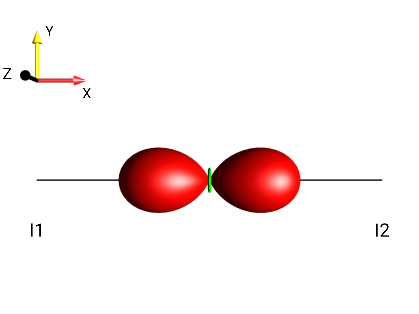Fig. 265 $$2I_{1x}I_{2x}$$¶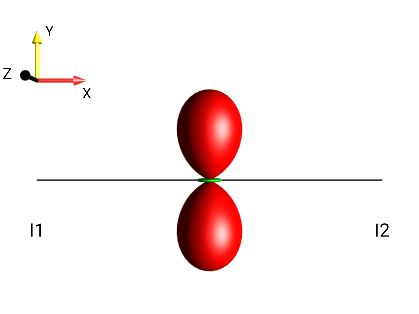Fig. 266 $$2I_{1y}I_{2y}$$¶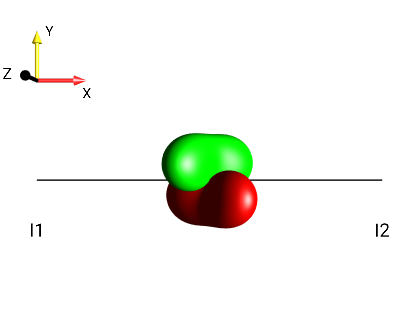Fig. 267 $$2I_{1x}I_{2z}$$¶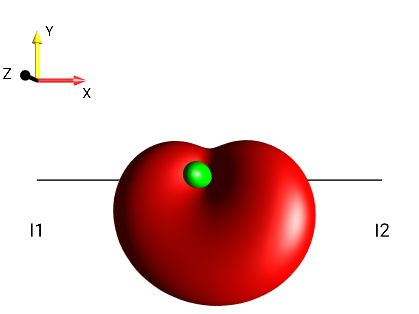Fig. 268 $$2I_{1x}I_{2x}+2I_{1y}I_{2y}+2I_{1x}I_{2z}$$¶

## How Can I Take a Screenshot of my DROPS Display on iOS?¶

A screen shot of the iPad or iPhone can be made by simultaneous clicking the home and power buttons.The screenshot will be saved in the Camera Roll. To see the screen shot, use the standard “Photos” app on the home screen.